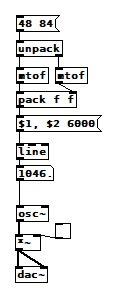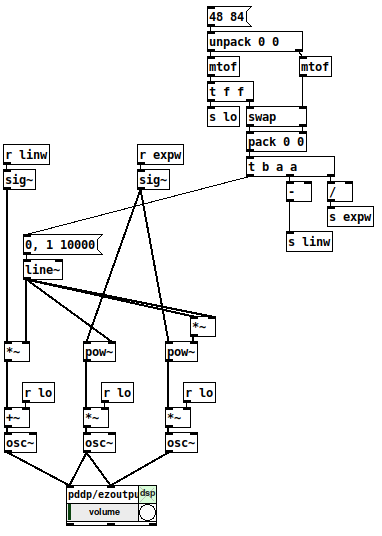• ### Logarithmic glissando

Hi, I'm stuck in a simple exercise from Loadbang book, which is : "Create a glissando that we hear as linear and one that we hear as logarithmic from C3 to C6." That's my functional linear version:However, about the logarithmic version (and other generalizations I can think about, like exponential, quadratic, etc...), is there an easier way than manually getting the parameters of a function like A*e^x + B and using expr?

• Posts 8 | Views 171
• @Cmaj7 I usually just use `[line~]` going into `[mtof~]`

• @Cmaj7 This should offer some guidance on making variable shaped curves. https://forum.pdpatchrepo.info/topic/12967/control-domain-waveforms
I really should update that abstraction, one of my first serious attempts, a little embarrassing in execution but it does the job. Works by doing the line in the 0 to 1 range and then scaling as needed so you can use [pow] to change the curve as just by tweaking the right inlet value, a power of 0.1 gives a square root curve, a power of 2 gives exponential and you can go higher or lower yet.

• in the logarithmic version you would move the mtof behind the line.

• Sorry to be a hair-splitter here, but the assignment in the OP clearly says:

"Create a glissando that we hear as linear and one that we hear as logarithmic from C3 to C6."

What we hear as a linear sweep is a log sweep: we perceive the same distance from A3 (220hz) to A4 (440hz) as we do from A4 (440hz) to A5 (880hz), while in reality the latter is twice as long as the former.

Hence, what we will hear as a linear sweep is @seb-harmonik.ar 's solution, while a linear upwards sweep like the one in OP's patch will sound as if it's slowing down exponentially - whether that qualifies as "hearing it as a logarithmic sweep" I will leave to the math geeks to ascertain

• it depends on what you treat as base. when you think in note numbers and not in hertz, it is the other way round.

but... we would have to distinguish between up and down. mtof wont do that of course.

(in my portamento abstraction the user may choose different settings for up and down individually. including "off"... )

• however the order will be like that: if you use a whatever scaling algorithm on the line output, the mtof still has to be on the end.• One of the very early lessons that I teach in my interactive multimedia class is range mapping, where I derive the formulas and then leave them with ready-to-use patch structures for them.

1. If it's based on incoming data, first normalize (0 to 1, or -1 to +1, range). If you're generating a control signal, generate a normal range (e.g. [phasor~] is already 0 to 1).

2. For both linear and exponential mapping, there's a low value `lo` and a high value `hi`. (Or, if the normalized range is bipolar -1 to +1, a center value instead of `lo`.)

3. The "width" of the range is: linear `hi - lo`, exponential `hi / lo`.

4. Apply the width to the control signal by: linear, multiplying (`(hi - lo) * signal`); exponential, raising to the power of the control signal = `(hi / lo) ** signal`.

5. Then (linear) add the lo or center; (exponential) multiply by the lo or center.

One way to remember this is that the exponential formula "promotes" operators to the "next level up": `+` --> `*`, `-` --> `/`, `*` --> power-of (and `/` --> log, but that would only be needed for normalizing arbitrary exponential data from an external source). So if you know the linear formula and the operator-promotion rule, then you have everything.

• linear: (width * signal) + lo
• exponential: (width ** signal) * lo

(Then the "super-exponential" that bocanegra was hypothesizing would exponentiate twice: `((width ** signal) ** signal) * lo` = `(width ** (signal * signal)) * lo`.)0723-triple-mapping-demo.pd

[mtof~] is a great shortcut, of course, but -- I drill this pretty hard with my students because if you understand this, then you can map any values onto any range, not only MIDI note numbers. IMO this is basic vocabulary -- you'll get much further with, say, western music theory if you know what is a major triad, and you can go much further with electronic music programming if you learn how to map numeric ranges.

hjh

Posts 8 | Views 171
Internal error.

Oops! Looks like something went wrong!